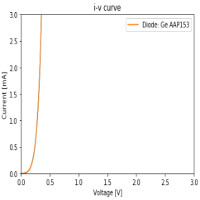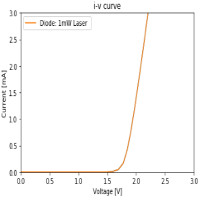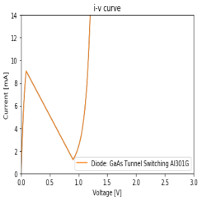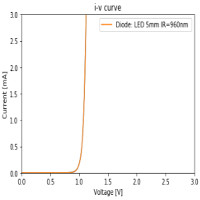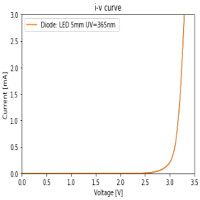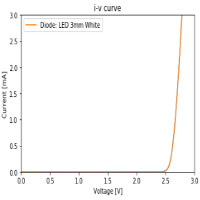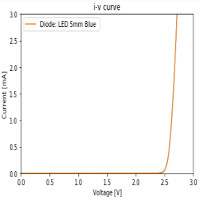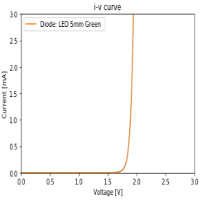I-V Curve: Diode A semiconductor diode is a two terminal nonlinear electronic device which conducts current predominantly in one direction. Due to it's nonlinearity a diode is one of the simplest examples of a device which does not follow Ohm's law. The simple I-V tracer built for the Ohm's law experiments [link] was tested for its ability to observe a 1N4148 diode's non-linearity (picture and scheme left) and plot its characteristic (Python code and graph below).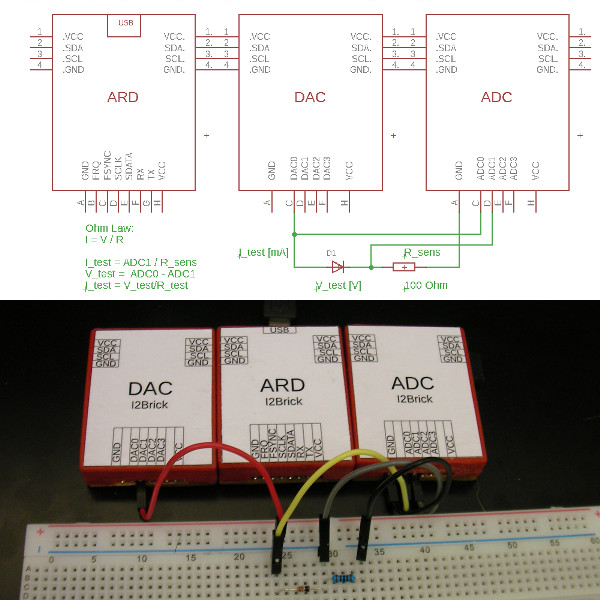In :
# Example Python code to demostrate ohm low.

from I2Brick import *
import matplotlib.pyplot as plt
%matplotlib inline

port = I2Brick('COM4')             #Windows: Initialize serial communication with I2Bricks
#port = I2Brick('/dev/ttyUSB0')    #Linux: Initialize serial communication with I2Bricks

print(ard(port))                   #test communication between ARD and PC

R_sense=100
voltage=[]
current=[]

for v in range (0, 4000,25):
dac(port,0, v)               #set dac voltage

port.close()
plt.plot(voltage, current)
plt.plot(voltage, current,label='Diode: 1N4148')
plt.axis([0,0.9,0,14])
plt.title('i-v curve')
plt.legend()
plt.xlabel('Voltage [V]')
plt.ylabel('Current [mA]')
plt.show()

I2Brick Firmware 3.0. Supported brick: ARD, OLD, DAC, ADC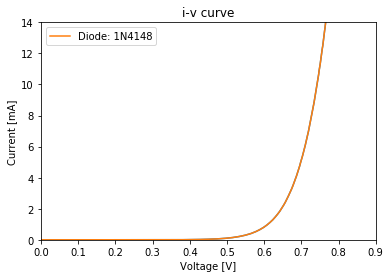DCA75 Pro Advanced Semiconductor Analyser For comparison, the 1N4148 was tested with the Peak Electronics Atlas DCA75 Pro Advanced Semiconductor Analyser (right).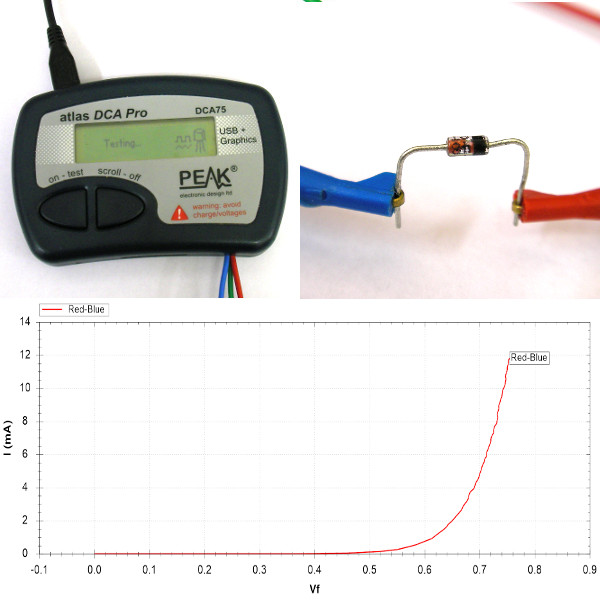There are more results for the simple I2Bricks I-V curve tracer controlled by Python code below.